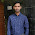## Control System Objective Questions From Competitive Exams

 A system is described by the following state and output equations

[dx1(t)/dt] =-3x1(t)+x2(t)+2u(t)
[dx2(t)/dt]=-2x2(t)+u(t)
y(t)=x1(t)
where u(t) is the input and y(t) is the output. The system transfer function is  [GATE 2009]
(a) (s+2)/(s2+5s-6)
(b) (s+3)/(s2+5s+6)
(c) (2s+5)/(s2+5s+6)
(d) (2s-5)/(s2+5s-6)

 A two-port network is defined by the relation: [IES 2010]

I=5V1+3V2
I2=2V1-7V2. The value of Z12 is
A. 3
B. -3
C. 3/41
D. 2/31

 The Z-transform of x(K) is given by
[IES 2010]

x(Z)= {(1-e-T)z-1} / {(1-z-1)(1-e-Tz-1)}
The initial value of x(0) is

A.  Zero
B.  1
C.  2
D.  3

  Consider the following statements with reference to the phase plane:
1.  They are general and applicable to a system of any order.
2.  Steady state accuracy and existence of limit cycle can be predicted.
3.  Amplitude and frequency of limit cycle if exists can be evaluated.
4.  Can be applied to discontinuous time system.
Which of the above statements are correct? [IES2010]
A. 1,2,3 and 4
B. 2 and 3 only
C. 3 and 4 only
D. 2,3 and 4 only

 For the circuit shown below, the natural frequencies at port 2 are given by s+2=0 and s+5=0,without knowing which refers to open-circuit and which to short-circuit. Then the impedences Z11 and Z22 are given respectively by [IES2010]
A.    K1{(s+5)/(s+2)}, K2{(s+2)/(s+5)}
B.    K1{(s+2)/(s+5)}, K2{(s+5)/(s+2)}
C.    K1{(s)/(s+2)}, K2{(s+2)/(s+5)}
D.    K1{(s+2)/(s+5)}, K2{(s+2)/(s+5)}

 Consider the following statements in connection with two-position controller:

1. If the controller has a 4% neutral zone,its positive error band will be 2% and negative error band will be 8%.
2. The neutral zone is also known as dead band.
3. The controller action of a two-position controller is very similar to that of a pure on-off controller.
4. Air-conditioning system works essentially on a two-position control basis.
Which of the above statements are correct? [IES2010]
A. 1,2 and 3 only.
B. 2,3 and 4 only.
C. 2 and 4 only.
D. 1,2,3 and 4

 The polar plot of an open loop stable system is shown below. The closed loop system is [GATE 2009]A. Always stable
B. Marginally stable
C. Unstable with one pole on the RH s-plane
D. Unstable with two poles on the RH s-plane

 The open loop transfer function of a unity feedback system is given by G(s) =(e-0.1s)/s.The gain margin of this system is
[GATE 2009]
A.  11.95 dB
B.  17.67 dB
C.  21.33 dB
D.  23.9 dB

9:01 PM

#### 1 comment:

1.Question No. 7 is unstable with 2 right hand poles because 2 clock wise encirclements are present